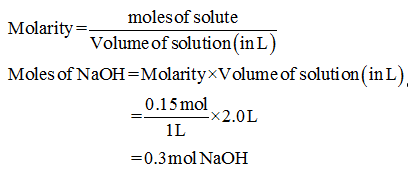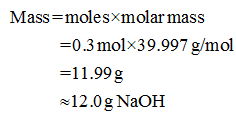# How do we prepare 2.0 L of a 0.15 M aqueous solution of sodium hydroxide, NaOH?

Question
1 views

How do we prepare 2.0 L of a 0.15 M aqueous solution of sodium hydroxide, NaOH?

check_circle

Step 1

Given,

Volume of solution = 2.0L.

Molarity of solution = 0.15M.

The moles of sodium hydroxide present in the 2.0L solution can be calculated asStep 2

The mass of sodium hydroxide in 0.3mol can be calculated as...

### Want to see the full answer?

See Solution

#### Want to see this answer and more?

Solutions are written by subject experts who are available 24/7. Questions are typically answered within 1 hour.*

See Solution
*Response times may vary by subject and question.
Tagged in

### Chemistry